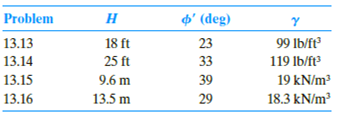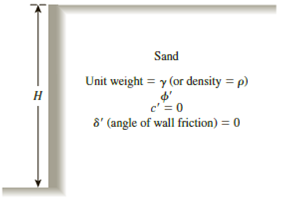Chapter 13, Problem 13.15PPrinciples of Geotechnical Enginee...

9th Edition
Braja M. Das + 1 other
ISBN: 9781305970939

Solutions

Chapter
SectionPrinciples of Geotechnical Enginee...

9th Edition
Braja M. Das + 1 other
ISBN: 9781305970939
Textbook Problem

13.13 through 13.16 Assume that the retaining wall shown in Figure 13.39 is frictionless. For each problem, determine the Rankine passive force per unit length of the wall, the variation of active earth pressure with depth, and the location of the resultant.Figure 13.39

To determine

Find the Rankine passive force σp(z=H) per unit length of the wall, the variation of active earth pressure Pa with depth, and the location z¯ of the resultant measured from the bottom of the wall.

Explanation

Given information:

The height (H) of the retaining wall is 9.6 m.

The soil friction angle ϕ is 39°.

The angle of wall friction δ is 0.

The unit weight γ of the soil is 19kN/m3.

Calculation:

Calculate the passive earth pressure coefficient (Kp) using the relation.

Kp=tan2(45+ϕ2)

Substitute 39° for ϕ.

Ka=tan2(45+39°2)=tan2(45+19.5)=tan2(64.5)=4.395

Calculate Rankine passive force σp(z=H) per unit length of the wall using the relation.

σp(z=H)=KpγH

Substitute 4.395 for Kp, 19kN/m3 for γ, and 9.6 m for H.

σp(z=H)=4

Still sussing out bartleby?

Check out a sample textbook solution.

See a sample solution

The Solution to Your Study Problems

Bartleby provides explanations to thousands of textbook problems written by our experts, many with advanced degrees!

Get Started

What are the basic characteristics of a NoSQL database?

Database Systems: Design, Implementation, & Management

How would you define artificial intelligence?

Principles of Information Systems (MindTap Course List)

Convert P = 5.00 atm into Pa, bar, and psia.

Fundamentals of Chemical Engineering Thermodynamics (MindTap Course List)

What is interpass temperature?

Welding: Principles and Applications (MindTap Course List)

Define the terms, intranet and extranet.

Enhanced Discovering Computers 2017 (Shelly Cashman Series) (MindTap Course List)